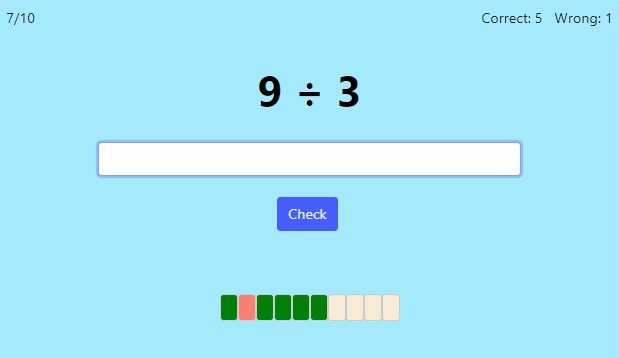MM Practice
×
Multiplication
Division
Place Value
Fractions & Decimals
Measurement
Statistics & Probability
Pre-Algebra
Money
Kindergarten
Geometry

# Basic Division FactsOnline practice for grades 3-5

This script allows you to practice any combination of the basic division facts (division tables) — either specific tables, such as division by 3 and by 5, or one of them (such as division by 8), or all of them (divisors up to 12). Very useful for 3rd and 4th grade students. 😃

You can choose timed or untimed practice, the number of practice problems, and which exact division tables you'd like to work on.

Screenshot:OR
Practice for a set time: min

Allow my comment to be posted on this site